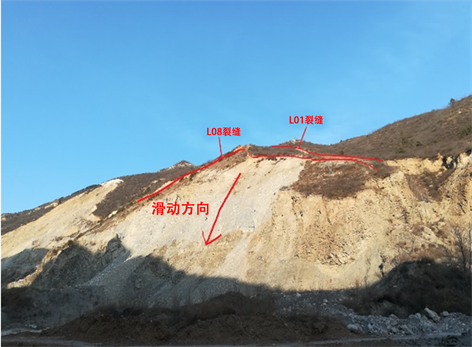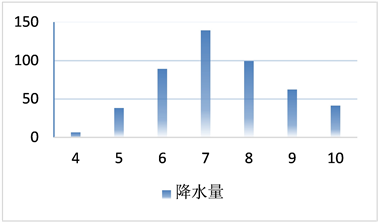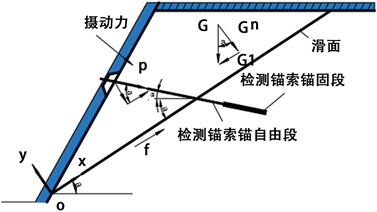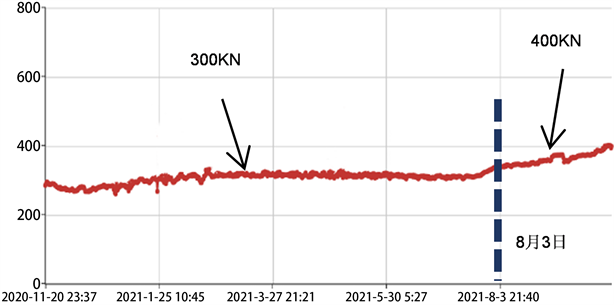#### 期刊菜单

Research on Slope Monitoring and Early Warning Method under Runoff-Seepage Conditions
DOI: 10.12677/HJCE.2022.114065, PDF , HTML, XML, 下载: 163  浏览: 207

Abstract: In order to study the influence of slope stability under rainfall conditions, a runoff-seepage model of landslides is established in this paper. In this model, the slope runoff is described by the Navier-Stokes equation, and the seepage is described by the Brinkman-extended Darcy equation. At the same time, the flow of the water body satisfies the mass conservation equation. According to the above equations, the flow velocity distribution characteristics in runoff and seepage can be deduced. Then Newton’s law of internal friction can be introduced to calculate the drag force generated by the water flow on the slope. Using this force, the formula for measuring the sliding force of the prestressed anchor cable is re-corrected. According to the sliding force measured on site, it can be judged that the slope is in the stage of instability and crack development. The results show that the formula has better applicability.

1. 引言

2. 工程概况Figure 1. Landslide overviewFigure 2. Average monthly rainfall

6~10月也为滑坡发生较多的月份，滑坡数占全年的90%以上，滑坡的发生时间与强降雨时间的分布非常契合。如2017年6月21日延庆区发布蓝色暴雨预警，每小时降雨量可达50 mm以上，同时边坡上发生了大规模的滑坡且对边坡下部支挡结构造成了严重的破坏，其具体情况如下图3所示。综上所述，降雨与滑坡灾害发生之间有紧密的关系，因此对降雨与滑坡的关系研究意义重大。

3. 流场分析及切应力计算

3.1. 应用模型

1) 边坡和径流可沿x方向无限延伸；

2) 边坡坡体介质均匀；

3) 水流为层流且仅在平面内运动；

4) 水体不可压缩，即满足连续性方程；

5) 径流流动可用Navier-Stokes方程进行描述；

6) 渗流可用Brinkman-extended Darcy方程进行描述 。Figure 4. Runoff analysis model

3.2. 径流的满足的方程

$\frac{\partial {u}_{x}}{\partial x}+\frac{\partial {u}_{y}}{\partial y}+\frac{\partial {u}_{z}}{\partial z}=0$

${f}_{x}-\frac{1}{\rho }\frac{\partial P}{\partial x}+v\left(\frac{{\partial }^{2}{u}_{x}}{\partial {x}^{2}}+\frac{{\partial }^{2}{u}_{x}}{\partial {y}^{2}}+\frac{{\partial }^{2}{u}_{x}}{\partial {z}^{2}}\right)=\frac{\partial {u}_{x}}{\partial t}+{u}_{x}\frac{\partial {u}_{x}}{\partial x}+{u}_{y}\frac{\partial {u}_{x}}{\partial y}+{u}_{z}\frac{\partial {u}_{x}}{\partial z}$

$\eta \frac{{\partial }^{2}{u}_{x}}{\partial {y}^{2}}+\frac{\Delta P}{L}+{\gamma }_{w}\mathrm{sin}\beta =0$

${u}_{x}=-\frac{\Delta P+{\gamma }_{w}L\mathrm{sin}\beta }{2\eta L}{y}^{2}+{A}_{1}y+{A}_{2}$

3.3. 边坡渗流的流速分布

$\frac{\partial {v}_{x}}{\partial x}+\frac{\partial {v}_{y}}{\partial y}+\frac{\partial {v}_{z}}{\partial z}=0$

$n\rho {f}_{x}-n\frac{\eta }{K}{v}_{x}-n\frac{\partial P}{\partial x}+\eta \left(\frac{{\partial }^{2}{v}_{x}}{\partial {x}^{2}}+\frac{{\partial }^{2}{v}_{x}}{\partial {y}^{2}}+\frac{{\partial }^{2}{v}_{x}}{\partial {z}^{2}}\right)=\frac{\rho }{n}\left({v}_{x}\frac{\partial {v}_{x}}{\partial x}+{v}_{y}\frac{\partial {v}_{x}}{\partial y}+{v}_{z}\frac{\partial {v}_{x}}{\partial z}\right)$

$\frac{{\text{d}}^{2}{v}_{x}}{\text{d}{y}^{2}}-n\frac{{v}_{x}}{K}+n\frac{\Delta P}{\eta L}+n\frac{{\gamma }_{w}\mathrm{sin}\beta }{\eta }=0$

${v}_{x}={C}_{1}{\text{e}}^{y\sqrt{n/K}}+{C}_{2}{\text{e}}^{-y\sqrt{n/K}}+\frac{\left(\Delta P+{\gamma }_{w}L\mathrm{sin}\beta \right)K}{\eta L}$

3.4. 边界条件

1) 在径流表面上，径流流速达到最大值，即当 $y=b+h$ 时，有 $\text{d}{u}_{x}/\text{d}y=0$

2) 在坡面处满足流速大小相等，即当 $y=b$ 时，有 ${u}_{x}={v}_{x}$

3) 在坡面处剪应力连续，即当 $y=b$ 时，有 $\frac{1}{n}\frac{\text{d}{v}_{x}}{\text{d}y}=\frac{\text{d}{u}_{x}}{\text{d}y}$

4) 在边坡底部渗流流速等于0，即当 $y=0$ 时， ${v}_{x}=0$

3.5. 切应力分析

$\tau =\eta \frac{\text{d}u}{\text{d}y}$

$\begin{array}{l}{\tau }_{x1}=\eta \left(2Ey+{A}_{1}\right)=-2E\eta \left(y-b-h\right)\\ \text{\hspace{0.17em}}\text{\hspace{0.17em}}\text{\hspace{0.17em}}\text{ }\text{\hspace{0.17em}}=-\frac{\Delta P+{\gamma }_{w}L\mathrm{sin}\beta }{L}\left(y-b-h\right)\end{array}$

4. 滑动力监测力学模型预警准则

4.1. 力学模型

$\begin{array}{c}{G}_{t}=P\left[\mathrm{cos}\left(\theta +\alpha \right)+\mathrm{sin}\left(\theta +\alpha \right)\mathrm{tan}\stackrel{¯}{\phi }\right]+G\mathrm{sin}\left(\theta +\alpha \right)\mathrm{tan}\stackrel{¯}{\phi }\\ \text{\hspace{0.17em}}\text{\hspace{0.17em}}-{\gamma }_{w}Lb\mathrm{cos}\left(\delta -\alpha \right)\mathrm{tan}\delta -{\gamma }_{w}h\left(\mathrm{tan}\delta +\mathrm{sin}\delta \right)\mathrm{cos}\left(\delta -\alpha \right)\end{array}$Figure 5. Landslide mechanics monitoring model

4.2. 监测数据分析Figure 6. No. 1 monitoring point dataFigure 7. No. 2 monitoring point data

5. 结论

  岑越, 刘振平, 刘建, 李强, 胡训健, 陈明. 奉节新铺滑坡裂缝位移与降雨天数相关性研究[J]. 水利水电技术(中英文), 2022, 53(2): 133-141.  彭思佳, 陈聪, 马金辉. 基于InSAR技术的矿区滑坡灾害形变诱因分析[J]. 科技创新与应用, 2022, 12(2): 75-77+82.  Tong, T.W. and Subramanian, E. (1985) A Boundary-Layer Analysis for Natural Convection in Vertical Porous Enclosures—Use of the Brinkman-Extended Darcy Model. International Journal of Heat & Mass Transfer, 28, 563-571. https://doi.org/10.1016/0017-9310(85)90179-6  杨进良. 土力学[M]. 第4版. 北京: 中国水利水电出版社, 2009: 76-77.  徐彤. 边坡变形监测研究与分析[J]. 科技创新与应用, 2022, 12(4): 142-144.  张文耀. 某露天煤矿边坡裂缝破坏机理及治理措施[J]. 同煤科技, 2021(6): 43-46.  丁辉. 边坡雷达在露天矿滑坡灾害预警中的应用[J]. 现代矿业, 2022, 38(1): 244-247+258.  饶奇, 蒋春宇. 基于可靠性理论的水电站大坝渗流风险识别方法[J]. 地下水, 2021, 43(6): 319-320.